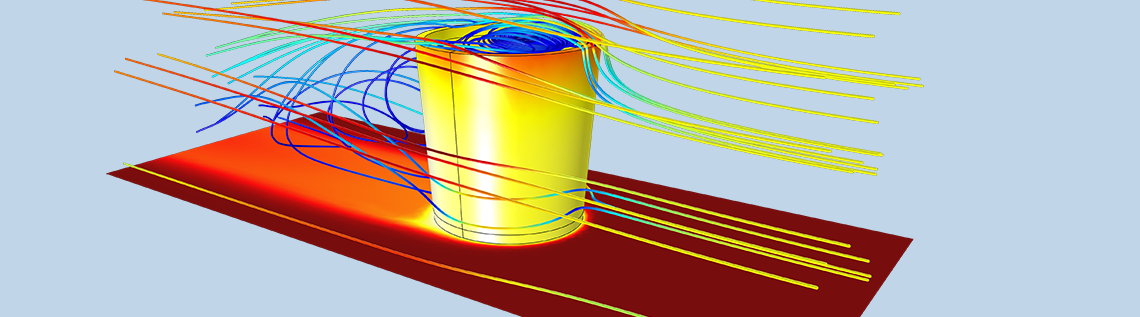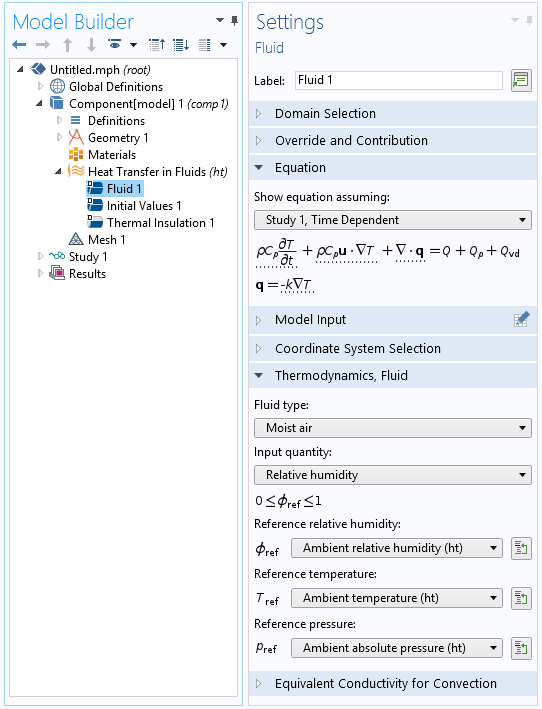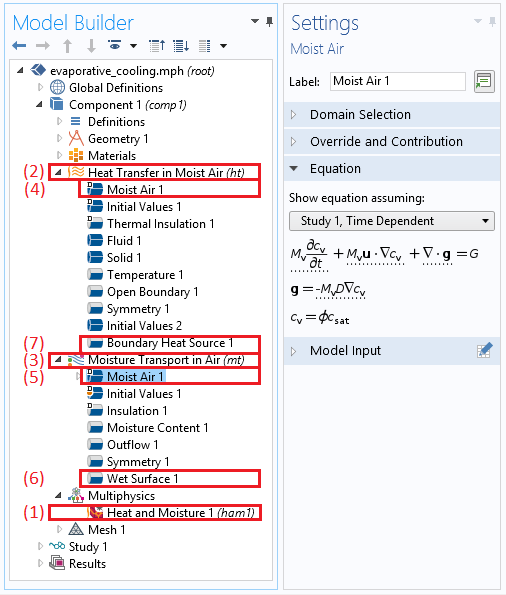# 如何使用 COMSOL® 模拟空气中的热湿传递

2017年 6月 9日

### 模拟湿空气传热

\phi=\frac{p_\textrm{v}}{p_\textrm{sat}(T)}

\rho C_p\frac{\partial T}{\partial t} + \nabla \cdot \left(- k \nabla T \right) +\rho C_p\textbf{u}\cdot\nabla T = Q

3 小时后的温度分布(左);3 小时后的相对湿度分布(中心);以及冷凝指示器变量，ht.condInd 随时间推移的演变(右图)。### 空气中热湿传递的耦合模拟

M_\textrm{v}\left(\frac{\partial c_\textrm{v}}{\partial t} + \nabla \cdot \left(- D \nabla c_\textrm{v} \right) +\textbf{u}\cdot\nabla c_\textrm{v}\right) = G

g_\textrm{evap} =M_\textrm{v}K(c_\textrm{sat}
-c_\textrm{v})

Q_\textrm{evap} =-L_\textrm{v}
g_\textrm{evap}

1. 热湿 耦合节点
2. 湿空气传热 接口
3. 空气中的水分输送 接口
4. 用于空气中热量传输的湿空气 特征
5. 用于空气中蒸汽输送的湿空气 特征
6. 用于液体表面蒸发的湿表面 特征
7. 将蒸发引起的潜热源添加到传热方程中的边界热源 特征### 自己尝试

• 查看此博客文章中介绍的教程模型：